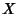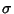## Sigma Algebra

Letbe a Set. Then a-algebrais a nonempty collection of Subsets ofsuch that the following hold:

1. The Empty Set is in.

2. Ifis in, then so is the complement of.

3. Ifis a Sequence of elements of, then the Union of thes is in.

Ifis any collection of subsets of, then we can always find a-algebra containing, namely the Power Set of. By taking the Intersection of all-algebras containing, we obtain the smallest such-algebra. We call the smallest-algebra containingthe-algebra generated by.

See also Borel Sigma Algebra, Borel Space, Measurable Set, Measurable Space, Measure Algebra, Standard Space